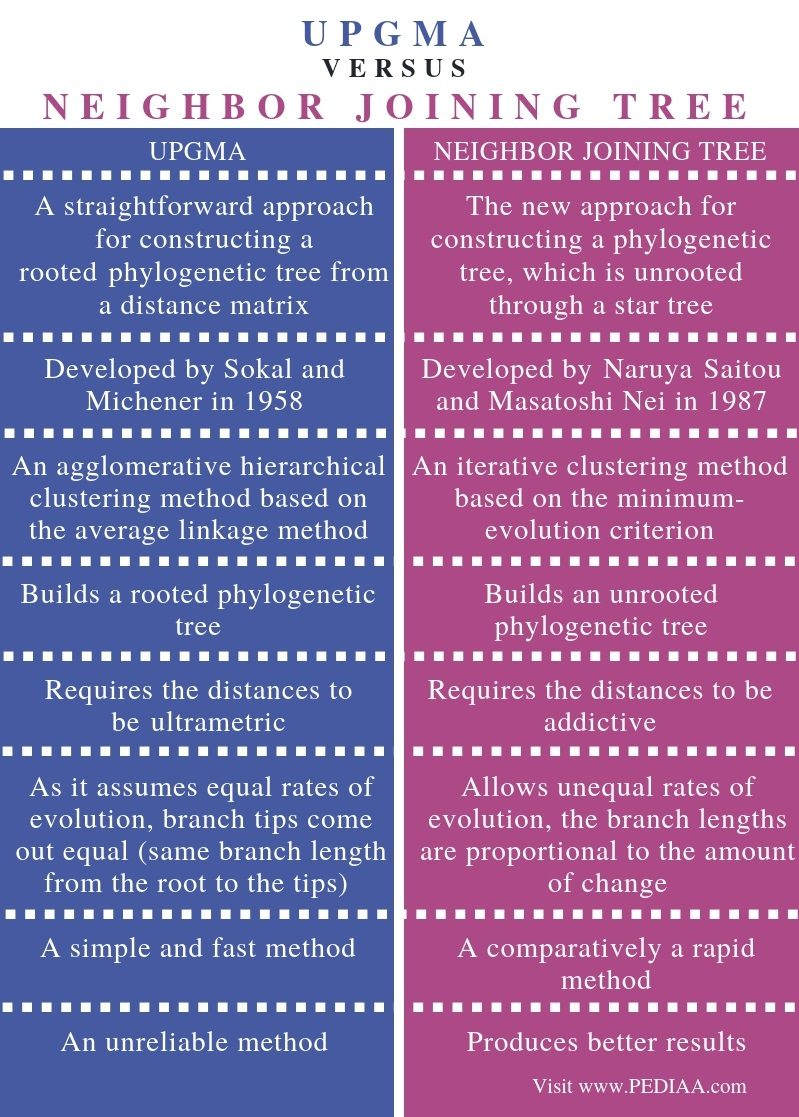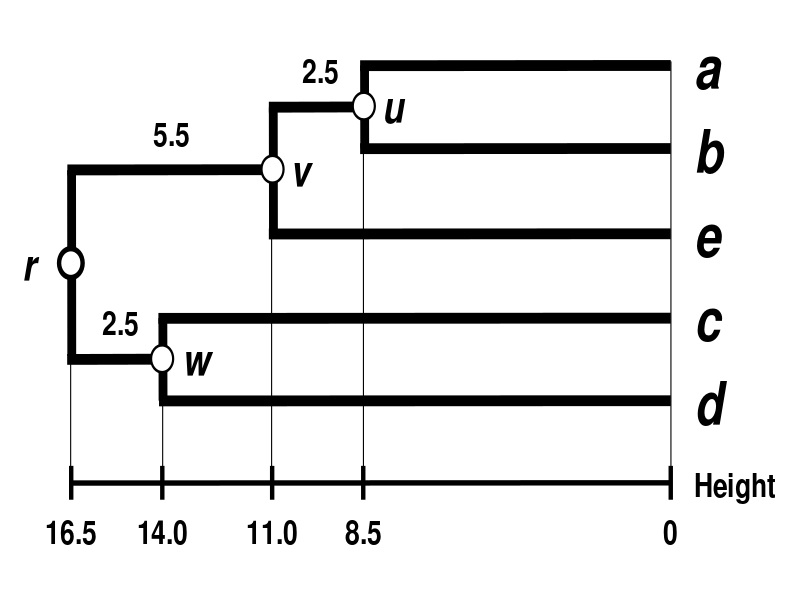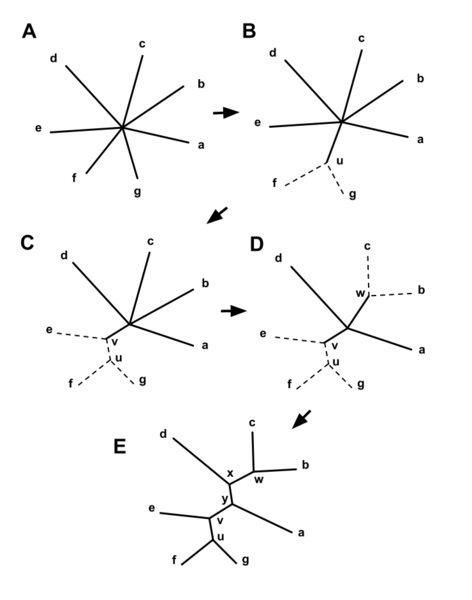# Difference Between UPGMA and Neighbor Joining Tree

The main difference between UPGMA and neighbor joining tree is that UPGMA is an agglomerative hierarchical clustering method based on the average linkage method whereas neighbor-joining tree is an iterative clustering method based on the minimum-evolution criterion. Furthermore, UPGMA produces a rooted phylogenetic tree while neighbor-joining tree method produces an unrooted phylogenetic tree. Since UPGMA method assumes equal rates of evolution, branch tips come out equal while as neighbor-joining tree method allows unequal rates of evolution, the branch lengths are proportional to the amount of change.

UPGMA (unweighted pair group method with arithmetic mean) and neighbor-joining (NJ) tree are the two types of algorithms, which build phylogenetic trees from a distance matrix. Generally, UPGMA is a simple, fast but, unreliable method while neighbor-joining tree method is a comparatively rapid method, giving better results when compared to the UPGMA method.

### Key Areas Covered

1. What is UPGMA
– Definition, Method, Significance
2. What is Neighbor Joining Tree
– Definition, Method, Significance
3. What are the Similarities Between UPGMA and Neighbor Joining Tree
– Outline of Common Features
4. What is the Difference Between UPGMA and Neighbor Joining Tree
– Comparison of Key Differences

### Key Terms

Agglomerative Clustering Methods, Distance Matrix, Neighbor-Joining Tree, Phylogenetic Tree## What is UPGMA

UPGMA (unweighted pair group method with arithmetic mean) is a simple, agglomerative, hierarchical clustering method attributed to Sokal and Michener. It is the simplest and fastest method for building a rooted and ultrametric phylogenetic tree. However, the major drawback of the method is its assumption of the same evolutionary rate on all lineages. This means the rate of mutations in these lineages is constant over time. This is also called the ‘molecular clock hypothesis’. In addition, it produces all the branches in the tree with similar distances. However, as it is difficult to have the same mutation rate for all lineages, in reality, the UPGMA method more often generates unreliable tree topologies.Figure 1: UPGMA Method

Furthermore, the UPGMA method starts with a matrix of pairwise distances. Initially, it assumes that each species is a cluster on its own. Then, it joins the closest two clusters with the smallest distance value in the distance matrix. Moreover, it recalculates the distance of the joint pair by taking the average. Then, the algorithm repeats the process until all species are connected in a single cluster.

## What is Neighbor Joining Tree

Neighbor-joining (NJ) tree method is the latest agglomerative clustering method used for building phylogenetic trees. It was developed by Naruya Saitou and Masatoshi Nei in 1987. However, it builds an unrooted phylogenetic tree. Moreover, it does not require ultrametric distances and uses the star decomposition method. Furthermore, the neighbor-joining tree algorithm adjusts for the variation of the evolutionary rates of lineages. Therefore, it begins with an unresolved star-like tree.Figure 2: Neighbor-Joining Tree Construction

Moreover, in the neighbor-joining tree method, the matrix Q is calculated based on the current distances. Then, it selects the pair of lineages with the lowest distance to join to a newly created node. However, this node is in a connection with the central node. After that, the algorithm calculates the distance from each lineage to the new node. Then it calculates the distance from each linage to the new node from the outside. Finally, it replaces the joined neighbors with the new node based on the calculated distances.

## Similarities Between UPGMA and Neighbor Joining Tree

• UPGMA and neighbor-joining tree are the two algorithms which build phylogenetic trees, taking a  distance matrix as the input. Generally, a distance matrix is a 2D matrix – an array that contains the pairwise distances of a set of points.
• The resulting alignment scores of a set of related protein or DNA sequences can be used as measures for the construction of the distance matrix.
• Both are agglomerative  (bottom-up)  clustering methods.
• They are faster methods which are computationally less expensive.
• Therefore, they can be applied in large data sets.
• Moreover, both methods produce better results when compared to the methods with other types of inputs.
• Although they are designed to produce single trees, sometimes they produce more than one topology, resulting in a ‘chaotic’ behavior based on the data-entering order.
• Bootstrap value is a simple statistical test to check the probability of nodes/clades formation.

## Difference Between UPGMA and Neighbor Joining Tree

### Definition

UPGMA refers to a straightforward approach for constructing a rooted phylogenetic tree from a distance matrix while neighbor-joining tree refers to the new approach for constructing a phylogenetic tree, which is unrooted through a star tree.

### Developed by

UPGMA method was developed by Sokal and Michener in 1958 while neighbor-joining tree was developed by Naruya Saitou and Masatoshi Nei in 1987.

### Significance

Moreover, UPGMA is an agglomerative hierarchical clustering method based on the average linkage method while neighbor-joining tree is an iterative clustering method based on the minimum-evolution criterion.

### Type of Phylogenetic Tree

While UPGMA method builds a rooted phylogenetic tree, neighbor-joining tree method builds an unrooted phylogenetic tree.

### Type of Distances

In addition, UPGMA algorithm requires the distances to be ultrametric while neighbor-joining tree algorithm requires the distances to be addictive.

### Nature of Branches of the Phylogenetic Tree

As UPGMA method assumes equal rates of evolution, branch tips come out equal (same branch length from the root to the tips). As neighbor-joining tree method allows unequal rates of evolution, the branch lengths are proportional to the amount of change.

### Speed

UPGMA is a simple and fast method while  neighbor-joining tree is a comparatively a rapid method.

### Reliability

Furthermore, UPGMA is an unreliable method while the neighbor-joining tree produces better results.

### Conclusion

UPGMA is one of the two algorithms to build a phylogenetic tree based on the evolutionary distance data. Moreover, it builds a rooted phylogenetic tree with similar branch lengths. In addition, it is the simple, fast, and the most reliable algorithm for building a phylogenetic tree from distance matrices. On the other hand, the neighbor-joining tree is the second method used to build a phylogenetic tree from a distance matrix. However, it produces an unrooted phylogenetic tree whose branch lengths reflect the amount of change during the evolution. Also, this algorithm builds the most reliable phylogenetic trees although the algorithm is comparatively less fast. Therefore, the main difference between UPGMA and the neighbor joining tree is the features of the phylogenetic tree and the features of the algorithm.

##### References:

1. Pavlopoulos, Georgios A et al. “A reference guide for tree analysis and visualization.” BioData mining vol. 3,1 1. 22 Feb. 2010, doi:10.1186/1756-0381-3-1
2. “UPGMA.” UPGMA Method, Available Here.
3. “Neighbor-Joining method.” Neighbor-Joining Method, Available Here

##### Image Courtesy:

1. “UPGMA Dendrogram 5S data” By Emmanuel Douzery. – Own work (CC BY-SA 4.0) via Commons Wikimedia
2. “Neighbor-joining 7 taxa start to finish” By Tomfy – Created with Google Docs drawing. (CC BY-SA 3.0) via Commons Wikimedia